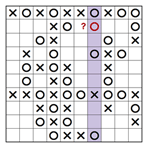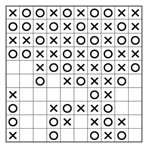﻿ Tic-Tac-Logic решение
 ВходРегистрация# Tic-Tac-Logic решение

Tic-Tac-Logic are single-player puzzles based on Tic-Tac-Toe, a game many of us used to enjoy as children. Each puzzle consists of a grid containing X's and O's in various places. The object is to place X or O in remaining squares so there are no more than two consecutive X's or O's in a row or a column, the number of X's is the same as the number of O's in each row and column, and all rows and all columns are unique. In the example below we have a 6x6 Tic-Tac-Logic puzzle with six rows and six columns. We now need to place X or O in the remaining squares according to the above rules… but how?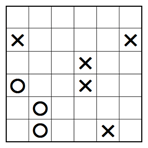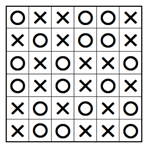## Basic techniques

Tic-Tac-Logic puzzles always start off with some very easy steps based on the first rule where no more than two consecutive X's or O's are allowed in a row or a column. More easy steps are then followed using the second rule where each row and column must contain an equal number of X's and O's, and the last rule where no two rows or two columns can be the same. Here are some ways of using the basic techniques:

#### 1. Avoiding triples 1

Most Tic-Tac-Logic puzzles start off with some given XX and OO doubles in various places. Since no more than two consecutive X's or O's are allowed in a row or a column, we can immediately block the doubles with an opposite symbol, one on each side, as shown in the example below.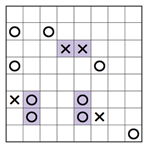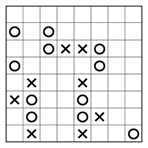#### 2. Avoiding triples 2

Another common situation is X_X and O_O where two symbols of the same type are separated by an empty square. Again, to avoid a forbidden triple we must place the opposite symbol in the empty square between as shown in the example below.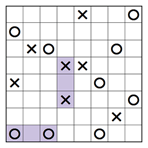#### 3. Avoiding triples 3

Sometimes X or O cannot be placed in a square because it will cause a forbidden triple one or two steps later. Let's look at row 6 of the partially solved puzzle below. If we place X in the left square then the two empty squares must contain O according to the rule each row and column must contain an equal number of X's and O's. However, this will create a triple OOO so the left square in row 6 must contain O.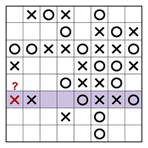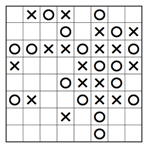#### 4. Completing a row or a column

According to the second rule, the number of X's and O's should be equal in each row and column. This means that in an 8x8 puzzle each row and each column should contain four X's and four O's. Looking at the highlighted row of the partially solved puzzle below we see four X's and only three O's so the last empty square must be O.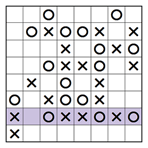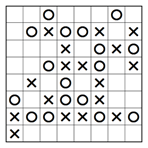#### 5. Avoiding row or column duplication

The third rule of Tic-Tac-Logic says that no two rows and no two columns can have the same sequence of X's and O's. In the partially solved puzzle below we see two highlighted columns, one completed and the other with two empty squares. Since the uncompleted column is missing one X and one O, there is only one way to place them without creating duplication as shown in the second image below.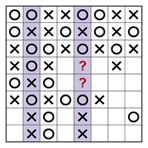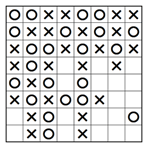The techniques described so far won't be enough to solve hard puzzles. For this you will need wits and advanced techniques to work out many special and interesting logic situations. Advanced techniques are based on a process of assumption and conflict, where a square is assumed to contain X (or O) and this assumption is proven wrong in the next step or two using logic. Here are some examples of advanced techniques to solve special situations. You will develop many more advanced techniques when you solve Tic-Tac-Logic puzzles by yourself: